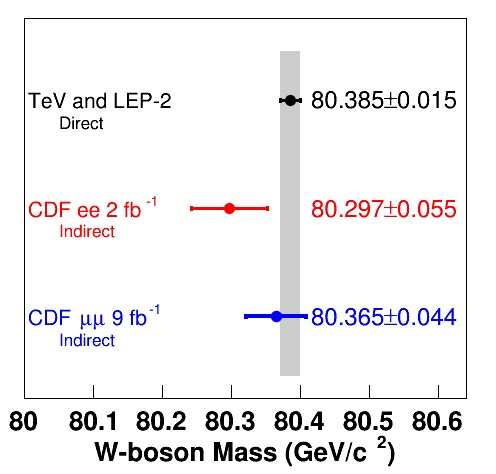# News & Events

August 15, 2013

#### Novel W-boson mass measurement provides a precise test of the Standard ModelIn the Standard Model of particle physics, the masses of the Z and Wbosons (MZ, MW) are related to another parameter of the theory, namely the electroweak mixing angle θW. The parameter sin2W) defines the relative strengths of the weak interaction (which causes radioactive decay) and the electromagnetic interaction. Since the Zboson decays into an electron and a positron, both of which can be tracked in the detector, the Z boson's mass is precisely determined. A precise measurement of the mass of the Wboson is harder because the W boson decays into an electron and a neutrino, which does not leave a trace in the detector and therefore goes undetected. Nonetheless, Rochester researchers have carried out precise measurements of the mass of the W boson at the Tevatron. In this experiment, a team led by Prof. Arie Bodek used Tevatron-CDF data to measure the mass of the W boson indirectly by measuring sin2W) and by using the Standard Model relation sin2W) = 1 - MW2/MZ2. A comparison of the indirect and direct measurements of the mass of the W boson provides a stringent test of the Standard Model.

The measurement uses a sample of 140,000 Z boson decays into electron-positron pairs, which were produced in proton-antiproton collisions at the Tevatron collider. Because of the interference between the weak interaction and the electromagnetic interaction in the Standard Model, on average the electron from the decay of the Z boson has a slight preference to be emitted in the direction of the proton. A measurement of the asymmetry between the number of Z events with electrons emitted in the direction of the proton and events for which the electrons are emitted in the direction of the antiproton can be related to the value of sin2W). The mass of the Wboson can thus be inferred from the precise measurement of sin2W).

The above figure shows a comparison of the world average of the combined direct measurements of the W mass fromCDFDZero and LEP-2 (labeled TeV and LEP-2) to our indirect measurement (labeled CDF ee 2 fb-1). The values of the direct and indirect measurements are in good agreement with each other, as expected in the Standard Model.

CDF physicists are now performing the analysis using the full Tevatron data set of 1.5 million Z decays. A new result with a much larger data sample is expected at the beginning of 2014.These CDF physicists contributed to this data analysis. From left: Arie Bodek, Jiyeon Han and Willis Sakumoto, all from the University of Rochester.